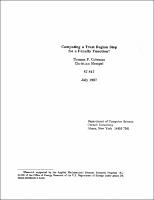## Computing a Trust Region Step for a Penalty Function##### Abstract
We consider the problem of minimizing a quadratic function subject to an ellipsoidal constraint when the matrix involved is the Hessian of a quadratic penalty function (i.e., a function of the form $p(x) = f(x) + \frac{1}{2\mu} c(x)^{T} c(x))$. Most applications of penalty functions require $p(x)$ to be minimized for values of $\mu$ decreasing to zero. In general, as $\mu$ tends to zero the nature of finite precision arithmetic causes a considerable loss of information about the null space of the constraint gradients when $\nabla^{2}p(x)$ is formed. This loss of information renders ordinary trust region Newton's methods unstable and degrades the accuracy of the solution to the trust region problem. The algorithm of More and Sorenson  is modified so as to be more stable and less sensitive to the nature of finite precision arithmetic in this situation. Numerical experiments clearly demonstrate the stability of the proposed algorithm.
1987-07
##### Publisher
Cornell University
##### Keywords
computer science; technical report
##### Previously Published As
http://techreports.library.cornell.edu:8081/Dienst/UI/1.0/Display/cul.cs/TR87-847
technical report